Search by Topic

Resources tagged with Maths Supporting SET similar to Odd One Out:

Filter by: Content type:
Age range:
Challenge level:

There are 93 results

Broad Topics > Applications > Maths Supporting SET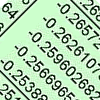Odd One Out

Age 16 to 18 Short Challenge Level:

In this short problem, can you deduce the likely location of the odd ones out in six sets of random numbers?Data Matching

Age 14 to 18 Challenge Level:

Use your skill and judgement to match the sets of random data.David and Goliath

Age 14 to 18 Challenge Level:

Does weight confer an advantage to shot putters?The Wrong Stats

Age 16 to 18 Challenge Level:

Why MUST these statistical statements probably be at least a little bit wrong?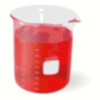Brimful 2

Age 16 to 18 Challenge Level:

Which of these infinitely deep vessels will eventually full up?Aim High

Age 16 to 18 Challenge Level:

How do you choose your planting levels to minimise the total loss at harvest time?Immersion

Age 14 to 16 Challenge Level:

Various solids are lowered into a beaker of water. How does the water level rise in each case?Global Warming

Age 14 to 16 Challenge Level:

How much energy has gone into warming the planet?Big and Small Numbers in the Living World

Age 11 to 16 Challenge Level:

Work with numbers big and small to estimate and calculate various quantities in biological contexts.Stirling Work

Age 16 to 18 Challenge Level:

See how enormously large quantities can cancel out to give a good approximation to the factorial function.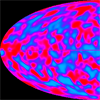Big and Small Numbers in Physics

Age 14 to 16 Challenge Level:

Work out the numerical values for these physical quantities.Approximately Certain

Age 14 to 18 Challenge Level:

Estimate these curious quantities sufficiently accurately that you can rank them in order of sizeBack Fitter

Age 14 to 16 Challenge Level:

10 graphs of experimental data are given. Can you use a spreadsheet to find algebraic graphs which match them closely, and thus discover the formulae most likely to govern the underlying processes?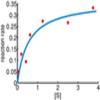Curve Fitter 2

Age 16 to 18 Challenge Level:

Can you construct a cubic equation with a certain distance between its turning points?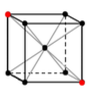Crystal Symmetry

Age 16 to 18 Challenge Level:

Use vectors and matrices to explore the symmetries of crystals.Investigating Epidemics

Age 11 to 16 Challenge Level:

Simple models which help us to investigate how epidemics grow and die out.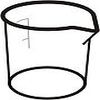Brimful

Age 16 to 18 Challenge Level:

Can you find the volumes of the mathematical vessels?Constantly Changing

Age 14 to 16 Challenge Level:

Many physical constants are only known to a certain accuracy. Explore the numerical error bounds in the mass of water and its constituents.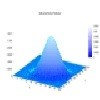Pdf Matcher

Age 16 to 18 Challenge Level:

Which pdfs match the curves?Fix Me or Crush Me

Age 16 to 18 Challenge Level:

Can you make matrices which will fix one lucky vector and crush another to zero?Vector Walk

Age 14 to 18 Challenge Level:

Starting with two basic vector steps, which destinations can you reach on a vector walk?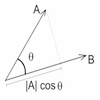Cross with the Scalar Product

Age 16 to 18 Challenge Level:

Explore the meaning of the scalar and vector cross products and see how the two are related.Scientific Curves

Age 16 to 18 Challenge Level:

Can you sketch these difficult curves, which have uses in mathematical modelling?Polygon Walk

Age 16 to 18 Challenge Level:

Go on a vector walk and determine which points on the walk are closest to the origin.Transformations for 10

Age 16 to 18 Challenge Level:

Explore the properties of matrix transformations with these 10 stimulating questions.Square Pair

Age 16 to 18 Challenge Level:

Explore the shape of a square after it is transformed by the action of a matrix.Matrix Meaning

Age 16 to 18 Challenge Level:

Explore the meaning behind the algebra and geometry of matrices with these 10 individual problems.Nine Eigen

Age 16 to 18 Challenge Level:

Explore how matrices can fix vectors and vector directions.Robot Camera

Age 14 to 16 Challenge Level:

Could nanotechnology be used to see if an artery is blocked? Or is this just science fiction?Pdf Stories

Age 16 to 18 Challenge Level:

Invent scenarios which would give rise to these probability density functions.Population Dynamics Collection

Age 16 to 18 Challenge Level:

This is our collection of tasks on the mathematical theme of 'Population Dynamics' for advanced students and those interested in mathematical modelling.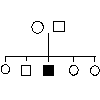Genetics

Age 14 to 16 Challenge Level:

A problem about genetics and the transmission of disease.How Do You React?

Age 14 to 16 Challenge Level:

To investigate the relationship between the distance the ruler drops and the time taken, we need to do some mathematical modelling...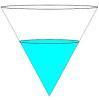Fill Me up Too

Age 14 to 16 Challenge Level:

In Fill Me Up we invited you to sketch graphs as vessels are filled with water. Can you work out the equations of the graphs?Designing Table Mats

Age 11 to 16 Challenge Level:

Formulate and investigate a simple mathematical model for the design of a table mat.Maths Filler 2

Age 14 to 16 Challenge Level:

Can you draw the height-time chart as this complicated vessel fills with water?Dangerous Driver?

Age 16 to 18 Challenge Level:

Was it possible that this dangerous driving penalty was issued in error?What's That Graph?

Age 14 to 16 Challenge Level:

Can you work out which processes are represented by the graphs?Building Approximations for Sin(x)

Age 16 to 18 Challenge Level:

Build up the concept of the Taylor seriesTaking Trigonometry Series-ly

Age 16 to 18 Challenge Level:

Look at the advanced way of viewing sin and cos through their power series.Scale Invariance

Age 16 to 18 Challenge Level:

By exploring the concept of scale invariance, find the probability that a random piece of real data begins with a 1.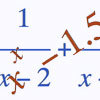Root Hunter

Age 16 to 18 Challenge Level:

In this short problem, try to find the location of the roots of some unusual functions by finding where they change sign.Differential Equation Matcher

Age 16 to 18 Challenge Level:

Match the descriptions of physical processes to these differential equations.Production Equation

Age 16 to 18 Challenge Level:

Each week a company produces X units and sells p per cent of its stock. How should the company plan its warehouse space?Spherical Triangles on Very Big Spheres

Age 16 to 18 Challenge Level:

Shows that Pythagoras for Spherical Triangles reduces to Pythagoras's Theorem in the plane when the triangles are small relative to the radius of the sphere.Over-booking

Age 16 to 18 Challenge Level:

The probability that a passenger books a flight and does not turn up is 0.05. For an aeroplane with 400 seats how many tickets can be sold so that only 1% of flights are over-booked?Integration Matcher

Age 16 to 18 Challenge Level:

Can you match the charts of these functions to the charts of their integrals?Air Routes

Age 16 to 18 Challenge Level:

Find the distance of the shortest air route at an altitude of 6000 metres between London and Cape Town given the latitudes and longitudes. A simple application of scalar products of vectors.Far Horizon

Age 14 to 16 Challenge Level:

An observer is on top of a lighthouse. How far from the foot of the lighthouse is the horizon that the observer can see?Perspective Drawing

Age 11 to 16 Challenge Level:

Explore the properties of perspective drawing.